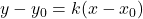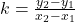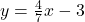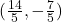Question

Find the points which divide the line segment joining the points (0, -3) and (7,1) in the ratio 2:3
internally and externally

1.Known two points, list function expression, useThe function expression isIt is known that the cross section of two points is 7, so the point of 2:3 is 14 / 5, that is, 0 + 14 / 5 is the abscissa of the point, which is substituted into the original equation to get y = – 7 / 5.

So the result is